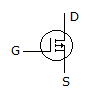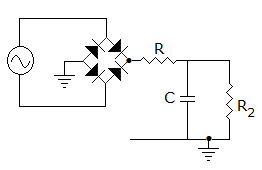# Online Electronics and Communication Engineering Test - Analog Electronics Test 1Loading Test...

Instruction:

• This is a FREE online test. DO NOT pay money to anyone to attend this test.
• Total number of questions : 20.
• Time alloted : 30 minutes.
• Each question carry 1 mark, no negative marks.
• DO NOT refresh the page.
• All the best :-).

1.

The quiescent collector current IC, and collector to emitter voltage VCE in a CE connection are the values when

A.
 ac signal is zeroB.
 ac signal is lowC.
 ac signal is negativeD.
 either (a) or (b)Explanation:

Quiescent-operation means operation when ac signal is not applied.

2.

A transistor has two p-n junctions. The batteries should be connected such that

A.
 both junctions are forward biasedB.
 both junctions are reverse biasedC.
 one junction is forward biased and the other is reverse biasedD.
 either (a) or (b)Explanation:

Emitter base junction is forward biased and base-collector junction is reverse biased.

3.

Assertion (A): In an op-amp the voltage gain and band width can be adjusted as per requirement

Reason (R): Large value capacitor can also be fabricated on a chip

A.
 Both A and R are correct and R is correct explanation for AB.
 Both A and R are correct but R is not correct explanation for AC.
 A is correct R is wrongD.
 A is wrong R is correctExplanation:

Large value capacitor cannot be fabricated on a chip.

4.

In a BJT amplifier the power gain from base to collector is 4000. The power gain in dB is

A.
 3.6B.
 36C.
 7.2D.
 72Explanation:

Gain in dB = 10 log (4000).

5.

In the diode circuit shown in figure the diodes are ideal. The impedance seen by source is

A.
 1 k ohmB.
 2 k ohmC.
 0.5 k ohmD.
 infiniteExplanation:

Only one diode conducts at one time.

6.

Typical values of current gains for CE, CB and CC amplifiers are

A.
 120, 120 and 1 respectivelyB.
 120, 1 and 120 respectivelyC.
 1, 120 and 120 respectivelyD.
 1, 1 and 120 respectively7.

The properties of FETs closely resemble those of a

A.
 thermionic valveB.
 P-N-P transistorC.
 N-P-N transistorD.
 UJT8.

Transformer coupling is mostly used in

A.
 RF amplifierB.
 audio amplifierC.
 both audio and RF amplifierD.
 none of the above9.

Symbol shown in figure represents aA.
 P-channel enhancement MOSFETB.
 N-channel DEMOSFETC.
 UJT with P-type baseD.
 UJT with N-type base10.

Which of the following statement about JFET is correct?

A.
 In an N-channel JFET, the channel is the narrowest under zero gate bias conditionB.
 In a JFET the gates are always forward biasedC.
 In a JFET, gate-source cut off voltage is numerically equal to the pinch off voltageD.
 It is a voltage controlled device11.

Primary advantage of a crystal oscillator is that

A.
 it can oscillate at any frequencyB.
 it gives a high O/P voltageC.
 its frequency of oscillation remains almost constantD.
 it operates on a very low dc supply voltage12.

The source of the harmonic distortion in a RC coupled transistor amplifier is usually

A.
 power supplyB.
 capacitorC.
 R-C circuitD.
 transistor13.

Which of the following uses a capacitor in the feedback path?

A.
 Active low pass filterB.
 Active high pass filterC.
 Both (a) or (b)D.
 Neither (a) nor (b)14.

Which of the following compensation method in amplifier can be used to reduce the bandwidth?

A.
 Pole-zero compensationB.
 Dominant pole compensationC.
 Lead compensationD.
 Any of the above15.

In figure, the function of resistor R isA.
 to reduce ripples in the outputB.
 to improve voltage regulationC.
 to limit the surge currentD.
 either (a) or (c)16.

A Colpitt's oscillator uses

A.
 a tapped inductorB.
 an inductor and two capacitorsC.
 both (a) and (b)D.
 either (a) or (b)17.

The gain of a source follower is unity.

A.
 TrueB.
 False18.

Which circuit has its output signal from, the emitter?

A.
 Common baseB.
 Common emitterC.
 Emitter followerD.
 None of the above19.

When the ac input signal is very large the instantaneous operating point CE amplifier moves all the way between

A.
 ac saturation and ac cut off pointsB.
 ac operating and ac cut off pointsC.
 ac saturation and ac operating pointsD.
 none of the above20.

The horizontal intercept of dc load line is the same as ideal

A.
 cut off pointB.
 saturation pointC.
 operating pointD.
 quasi saturation point